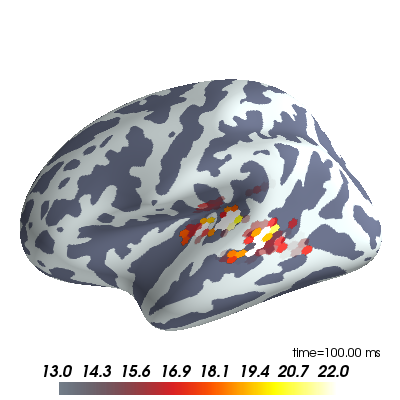# Plot MEG inverse solution¶

Data were computed using mne-python (http://martinos.org/mne)

```import os
import numpy as np

from surfer import Brain

print(__doc__)
```

Set up some useful variables and make the plot.

```# define subject, surface and hemisphere(s) to plot:

subject_id, surf = 'fsaverage', 'inflated'
hemi = 'lh'

# create Brain object for visualization
brain = Brain(subject_id, hemi, surf, size=(400, 400), background='w',
interaction='terrain', cortex='bone', units='m')

# label for time annotation in milliseconds

def time_label(t):
return 'time=%0.2f ms' % (t * 1e3)

# Read MNE dSPM inverse solution and plot

for hemi in ['lh']:  # , 'rh']:
stc_fname = os.path.join('example_data', 'meg_source_estimate-' +
hemi + '.stc')

# data and vertices for which the data is defined
data = stc['data']
vertices = stc['vertices']

# time points (in seconds)
time = np.linspace(stc['tmin'], stc['tmin'] + data.shape * stc['tstep'],
data.shape, endpoint=False)

# colormap to use
colormap = 'hot'

# add data and set the initial time displayed to 100 ms,
# plotted using the nearest relevant colors
smoothing_steps='nearest', time=time, time_label=time_label,
hemi=hemi, initial_time=0.1, verbose=False)

# scale colormap
brain.scale_data_colormap(fmin=13, fmid=18, fmax=22, transparent=True,
verbose=False)
```To change the time displayed to 80 ms uncomment this line:

```# brain.set_time(0.08)
```

uncomment these lines to use the interactive TimeViewer GUI

```# from surfer import TimeViewer
# viewer = TimeViewer(brain)
```

Total running time of the script: ( 0 minutes 0.734 seconds)

Gallery generated by Sphinx-Gallery